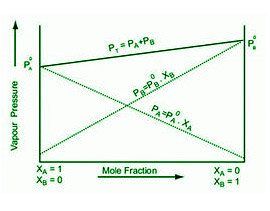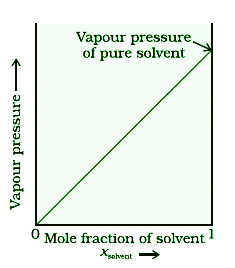GFG App
Open AppBrowser
Continue

# Vapour Pressure of Liquid Solutions

When a liquid is allowed to evaporate in a closed vessel, some of the liquid evaporates and the vapours fill the remaining space. Since the vapours leave the container, they condense above the liquid’s surface in a vapour state. Liquid transforms into vapours as a result of vaporisation, and the volume of the liquid decreases. The number of gaseous molecules in the vapour phase continuously increases as evaporation progresses. These molecules wander around at random in a confined space, and some of them collide with the surface of a liquid and condense. The process of condensation works in the opposite direction of evaporation. As a result, both evaporation and condensation take place at the same time.

### Vapour pressure of liquid solutions

At a given temperature, the vapour pressure of a liquid/solution is the pressure exerted by vapours in equilibrium with the liquid/solution.

Vapour pressure α escaping tendency

Vapour pressure of liquid solutions and Raoult’s Law (Raoult’s law for volatile solutes)

The partial vapour pressure of each component in a solution of volatile liquids is precisely proportional to its mole fraction, according to Raoult’s law.

Consider a solution containing two volatile components 1 and 2, each with a mole fraction of x1 and x2. Assume their partial vapour pressures are p1 and p2, respectively, at a certain temperature, and their pure state vapour pressures are p10 and p20. As a result, for component 1, according to Raoult’s law,

p1  α  x1

and

p1 = p10 x1

Similarly, for component 2

p2 = p20 x2

The total pressure (ptotal) over the solution phase in the container, according to Dalton’s partial pressure law, is equal to the sum of the partial pressures of the solution’s components and is given as :

ptotal = p1 + p2

substituting the values of p1 and p2, we get

ptotal = x1p10 + x2p20

= ( 1 – x2 )p10 + x2p20

= p10 + ( p20 – p10 )x2

At constant temperature, the plot of vapour pressure and mole fraction of an ideal solution. The partial pressures of the components are shown by the dashed lines I and II. The graphic clearly shows that p1 and p2 are proportional to x1 and x2, respectively. The total vapour pressure is represented by line III in the diagram.• Mole fraction in the vapour phase

Using Dalton’s rule of partial pressures, if y1 and y2 are the mole fractions of components 1 and 2 in the vapour phase, then:

p1 = y1ptotal

p2 = y2ptotal

In general,

pi = yiptotal

• Conclusions on the Relationship between Vapour Pressure and Liquid-Liquid Solutions

The following conclusions can be drawn from the relationship. The mole fraction of any one of the components, A or B, determines total vapour pressure. The equation can alternatively be written as, for example,

Ptotal = PB0 + (PA0 – PB0) XA

Any of the following components can be plotted as a linear graph between total pressure and mole fraction. Depending on the pure vapour pressures of components A and B, total vapour pressure increases or drops with regard to the mole fraction of component A.

The total vapour pressure can be determined if the components are in vapour form.

PA = yA Ptotal

PB = yB Ptotal

The mole fractions of components A and B in the vapour phase are yA and yB, respectively.

• As a Special Case of Henry’s Law, Raoult’s Law

One of the components in a binary liquid-liquid solution is sufficiently volatile that it exists as a gas whose solubility is regulated by Henry’s Law. The partial pressure of a gas in the vapour phase is proportional to the mole fraction of the gas in the solution, according to Henry’s Law, and is stated as P = KHX

The partial pressure of the solution is P, and the mole fraction of the solvent is X. The proportionality constant (Henry’s constant) is denoted by KH.

The Raoult’s Law is defined as follows:

P = P0 X

The partial pressure of the solution is P, and the mole fraction of the solvent is X. P0 denotes the pure solvent’s vapour pressure. When the equations of Henry’s law and Raoult’s law are compared, we get:

KH = P0

As a result, Raoult’s Law is a subset of Henry’s Law.

Vapour pressure of solutions of solids in liquids and Raoult’s Law

When a non-volatile solute is added to a solvent to make a solution, the number of solvent molecules escaping from the surface decreases, lowering the vapour pressure. The amount of non-volatile solute present in the solution, regardless of its composition, influences the decrease in vapour pressure of the solvent. In its most general form, Raoult’s law states that the partial vapour pressure of each volatile component in a solution is directly proportional to its mole fraction for every solution.

Let’s call the solvent 1 and the solute 2 in a binary solution. Only the solvent molecules are present in the vapour phase when the solute is non-volatile, and they contribute to the vapour pressure. Let p1 denote the solvent’s vapour pressure, x1 its mole fraction, and p10 its vapour pressure in the pure state. Then, based on Raoult’s law,

p1  α  x1

and p1 = x1 p10 = ptotalWhen a non-volatile solute is added to a solvent, why does the vapour pressure drop?

The following factors contribute to the drop in vapour pressure :

• Because evaporation is a surface process, the more surface area, the more evaporation and thus the higher the vapour pressure. In a pure liquid, the molecules have a greater surface area to vaporize, resulting in higher vapour pressure. When a non-volatile solute is added, however, the solvent molecules have less surface area to escape, resulting in low vapour pressure.
• The number of molecules evaporating or leaving the surface is substantially bigger in pure liquid solutions than that of non-volatile solute insolvent
• Consider a solution in which A is the solvent and B is the solute to determine the overall vapour pressure of the solid-liquid solution. We know that the partial vapour pressure of a single component (solute/solvent) is directly proportional to its mole fraction thanks to Raoult’s law.
• When we add a non-volatile solute, it’s clear that the only source of vapour pressure will be the solvent, because it’s the only component available in the vapour phase. As a result, if PA is the solvent’s vapour pressure, xA is its mole-fraction, and PA0 is the pure solvent’s vapour pressure, Raoult’s law yields the following relationship:

PA  α  xA

PA = PA0 xA

When we plot a graph between the mole fraction of solvent and the vapour pressure, we see that it is linear in character.

### Ideal and Non – ideal solutions

Ideal Solutions: An ideal solution is one in which each component follows Raoult’s rule under all temperature and concentration circumstances.

Properties of Ideal solutions-

• ∆HMIXING = 0
• ∆VMIXING = 0
• Intermolecular attractive forces between the A – A and B – B are nearly equipments to those between A – B.

Examples are the Solution of benzene and toluene, a Solution of n-hexane and n-heptane.

Non-Ideal Solutions: The term “non-ideal solution” refers to a solution that does not obey Raoult’s law over the whole concentration range.

• Solutions showing positive deviation from Raoult’s law-
• Solvent-Solute (A – B) type of force is weaker than Solute-Solute (B – B) and Solvent- Solvent (A – A) forces.
• The vapour pressure is higher than the law predicts.
• ∆HMIXING > 0
• ∆VMIXING > 0

Examples: Ethanol and acetone, Carbon disulphide and acetone.

• Solutions showing negative deviation from Raoult’s law-
• Solvent-Solute (A – B) type of force is stronger than Solute-Solute (B – B) and Solvent- Solvent (A – A) forces.
• The vapour pressure is lower than the law predicts.
• ∆HMIXING < 0
• ∆VMIXING < 0

Examples: Phenol and aniline, chloroform and acetone etc.

### Sample Questions

Question 1: What effect does temperature have on a solution’s vapour pressure?

A liquid’s vapour pressure is directly proportional to its temperature, i.e. it rises as the temperature rises. This is because when the temperature rises, more molecules will have higher kinetic energies. As a result, more molecules will escape from the liquid’s surface into the vapour phase, resulting in a higher vapour pressure.

Question 2: Pure chloroform CHCl3 and dichloromethane CH2Cl2 have vapour pressures of 200 mm Hg and 415 mm Hg, respectively. What is the total vapour pressure of a solution containing 30 g of CHCl3 and 42 g of CH2Cl2 at a normal temperature of 298 K? In the vapour phase, determine the mole fraction of both components.

Mole fraction of component A = moles of A / Total moles of the solution

And Moles of component = mass of component/ molar mass of component

CHCl3 = 30 / 119.5 = 0.25

CH2Cl2 = 42 / 85 = 0.49

Let A component be CHCl3 and B component be CH2Cl2 , then

xA = 0.25 / ( 0.25 + 0.49) = 0.25 / 0.74 = 0.33

xB = 0.49 / ( 0.25 + 0.49) = 0.49 / 0.74 = 0.66

Also, we have PA0 as 200 mm Hg and PB0 as 415 mm Hg

Then total vapour pressure P equals

Ptotal = PA0 xA + PB0 xB

Ptotal = 200 × 0.33 + 415 × 0.66

Ptotal = 339.9 mm Hg

In the vapour phase, let yA be the mole fraction of CHCl3 chloroform and yB be the mole fraction of CH2Cl2.

PA = yA Ptotal

PA0 xA = yA Ptotal

200 × 0.33 = yA Ptotal

66 / 339.9 = yA

0.19 = yA

Also, yA + yB = 1

Then yB = 1 – yA = 1 – 0.19 = 0.81

As a result, the solution’s total vapour pressure is 339.3 mm Hg, and the mole fractions of CHCl3 chloroform and CH2Cl2 dichloromethane are 0.19 and 0.81, respectively.

Question 3: Does the type of the non-volatile solute affect the vapour pressure of a solution?

The type of the non-volatile solute has no bearing on the vapour pressure of a liquid. It is, however, dependent on the amount of non-volatile solute used. For example, adding 1.0mol of glucose to one kilogramme of water reduces the vapour pressure roughly identically to adding 1.0mol of urea to the same amount of water at the same temperature.

Question 4: How do you calculate a liquid’s vapour pressure?

Raoult’s law can be used to calculate a liquid’s vapour pressure. The following is the expression supplied by Raoult’s law:

P1 = P10 X1

Where P01 is the pure vapour pressure of the solvent, P1 is the vapour pressure of a solution containing a non-volatile solute and a volatile solvent, and X1 is the solvent’s mole fraction.

Question 5: Which one has the highest vapour pressure?